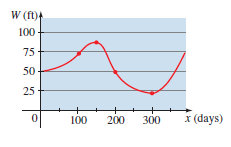# The intervals on which the function W is increasing and decreasing.### Precalculus: Mathematics for Calcu...

6th Edition
Stewart + 5 others
Publisher: Cengage Learning
ISBN: 9780840068071### Precalculus: Mathematics for Calcu...

6th Edition
Stewart + 5 others
Publisher: Cengage Learning
ISBN: 9780840068071

#### Solutions

Chapter 2.3, Problem 47E

(a)

To determine

## To find: The intervals on which the function W is increasing and decreasing.

Expert Solution

The intervals on which the function W is increasing is [0,150][300,) and decreasing on [150,300] .

### Explanation of Solution

Given information:

The graph shows the depth of water W in reservoir over a one-year period as a function of the number of days x since the beginning of the year.Calculation:

As observed from the graph, the depth of water increases in first 150 days and then decreases for 150 days and then increases for the rest of the days.

So, the function W increases from 0 to 150 , decreases from 150 to 300 and it increases again from 300 onwards.

Therefore, the intervals on which the function W is increasing is [0,150][300,) and decreasing on [150,300] .

(b)

To determine

### To find: The value of x for which W achieve a local maximum and local minimum.

Expert Solution

The value of x for which W achieve a local maximum is x=150 and local minimum is x=300 .

### Explanation of Solution

Given information:

The graph shows the depth of water W in reservoir over a one-year period as a function of the number of days x since the beginning of the year.Calculation:

As observed from the graph, the function achieves the maximum depth of water at 100 days and it achieves the minimum depth of water at 300 days.

So, the function W has a maximum value at x=100 and minimum value at x=300 .

Therefore, the value of x for which W achieve a local maximum is x=150 and local minimum is x=300 .

### Have a homework question?

Subscribe to bartleby learn! Ask subject matter experts 30 homework questions each month. Plus, you’ll have access to millions of step-by-step textbook answers!